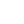----------- Your trusted source for independent sensor data- Photons to Photos------------ Last revised: 2021-06-12 03:00 GMT-5

# --------------- Optics Primer - Light Throughput with a Close-up Lens

--------------------------------------------------------- By Bill Claff

Recall that for light throughput we confirmed conservation of light between the object and image sides of the lens and that the solid angle incorporates the half-angle of view.
Etendue can be expressed as:

G = (π / 2* (Y / ef#))2 Where ef# is the effective f#.

And buried in ef# is our usual (1 + m / p) factor; which we will see again shortly.

Close-up photographers often speak of light "loss" and explain it in terms of the inverse-square law and the increased distance of the exit pupil from the image plane when focusing closer than infinity.
We can confirm this behavior by inspecting the image circle of a bare lens.

I prefer to think of the optical system as constrained by Y, the actual image height; and that the image circle is defined by this constrained image plane.
So rather than  light loss I think of it in terms of less light gathered. This doesn't change the outcome, it's just a different point of view.

In this regard, it's helpful to revisit the formula for the half-angle of view (which is a function in part of Y):

tan(wO) = (Y / f) * / (1 + m / p)

Note that the angle goes down as m is increased; and that the angle goes up as f is decreased.
Viewed this way it is easier to understand how a close-up lens might lose less light than extension tubes due to the drop in focal length which somewhat offsets the increase in magnification.
In fact, perhaps no light is lost, or even, light is gained.

When a close-up lens is attached the new focal length is determined by the power formula:

P = PM + PC - d * PM * PC
where P is power in diopters, subscript M indicates main lens, subscript C indicates close-up lens, and d is the distance between principal planes in meters.

Although it is seldom true we will assume that d is zero. This is a very common assumption which is rarely stated.

Since P = 1000mm / f we can convert from P = PM + PC to focal lengths:

f = fM * fC / (fM + fC)

Let's call the ratio of the focal length term Rf:

Rf = f / fM = (fM * fC / (fM + fC)) / fM = fC / (fM + fC)

Our assumption implies that the rear principal plane doesn't move resulting magnification is:

m =  (1 + mM) * fM / f - 1

Now, we have a bit of algebra ahead of us. Let's first substitute for f, eliminate fM, and substitute Rf:

m = (1 + mM) * fM * (fM + fC)/ (fM * fC) - 1 = (1 + mM) * (fM + fC)/ fC - 1 = (1 + mM) / Rf - 1

The magnification term in the half-angle formula is:

(1 + m / p) = (p + m) / p

Let's call the ratio of the magnification term Rm:

Rm = ((p + (1 + mM) / Rf - 1) / p) / ((p + m) / p) = (p + (1 + mM) / Rf - 1) / (p + mM)

Let's call the product of Rf and Rm the light throughput ratio, Rg:

Rg = Rf * Rm

So we substitute for Rm and simplify:

Rg = Rf * Rm = Rf * (p + (1 + mM) / Rf - 1) / (p + mM) = Rf * p + (1 + mM) - Rf) / (p + mM)

To understand whether light is lost or gained we want to know how Rg compares to 1:

1 Rg      where indicates an as yet unknown relationship

We insert our formula for Rg and cross-multiply:

(p + mM) Rf * p + (1 + mM) - Rf = Rf * (p - 1) + (1 + mM)

And then eliminate mM from each side:

p Rf * (p - 1) + 1

It's not surprising that initial magnification falls out and that as a sanity check when Rf is 1 we get p = p

Finally, rearrange with p on the left hand side:

p * (1 - Rf) (1 - Rf)

and (whew):

p 1

### Conclusion

Remember that we made an assumption about the close-up lens mating perfectly with the main lens.
With that caveat we have determined that no light is lost if the main lens p (pupil magnification) is unity, light is gained if p is > 1, and light is lost if p < 1.

In practice close-up lenses are normally used with lenses that have pupil magnifications less than unity so in these circumstances light is in fact lost.
However, we have seen earlier that less light is lost than using extension to get to the same magnification with the trade-off being less working distance with a close-up lens.